SHARE
HELP

To start with, Sr2Jr’s first step is to reduce the expenses related to education. To achieve this goal Sr2Jr organized the textbook’s question and answers. Sr2Jr is community based and need your support to fill the question and answers. The question and answers posted will be available free of cost to all.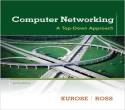# Computer Networking : A Top-down Approach

Authors:
James F. Kurose, Keith W. Ross
Exercise:
Problems
Chapter:
Multimedia Networking
Edition:
6
ISBN:
9780132856201
Question:
19
 Previous Next

###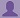Question

Consider again the figure for P18.

a. Assume a priority service, with packets 1, 4, 5, 6, and 11 being high-priority packets. The remaining packets are low priority. Indicate the slots in which packets 2 through 12 each leave the queue.

b. Now suppose that round robin service is used, with packets 1, 4, 5, 6, and 11 belonging to one class of traffic, and the remaining packets belonging to the second class of traffic. Indicate the slots in which packets 2 through 12 each leave the queue.

c. Now suppose that WFQ service is used, with packets 1, 4, 5, 6, and 11 belonging to one class of traffic, and the remaining packets belonging to the second class of traffic. Class 1 has a WFQ weight of 1, while class 2 has a WFQ weight of 2 (note that these weights are different than in the previous question). Indicate the slots in which packets 2 through 12 each leave the queue. See also the caveat in the question above regarding WFQ service.

###Answer

a)

Consider the figure P18 and take a priority service, with packets 1, 4, 5, 6, and 11 being high-priority packets and remaining are low priority packets.

The following table indicating the arrival time and the time at which the each packet leaves the queue.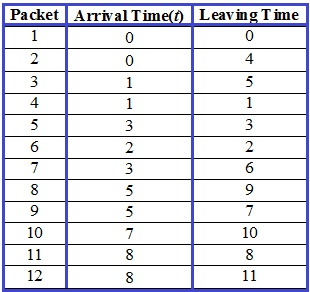The formula for the Delay time  = leaving time – arrival time.

The following table calculate the delay time of the packets by using above formula: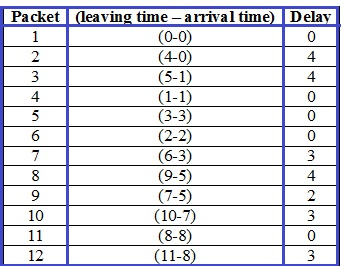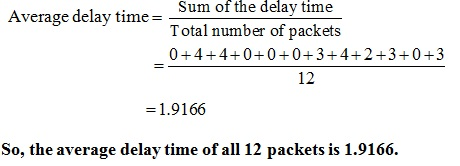b)

Consider the round robin service is used, with packets 1, 4, 5, 6, and 11 belonging to one class of traffic, and the remaining packets belonging to the second class of traffic.

The following table indicating the arrival time and the time at which the each packet leaves the queue.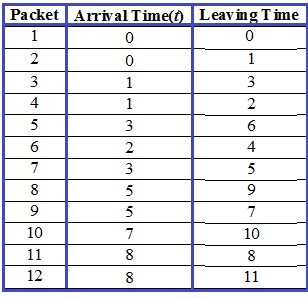The formula for the Delay time  = leaving time – arrival time.

The following table calculate the delay time of the packets by using above formula: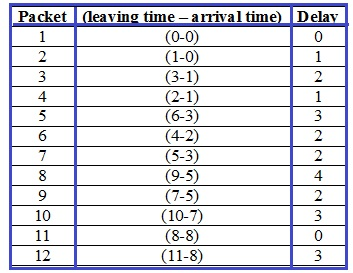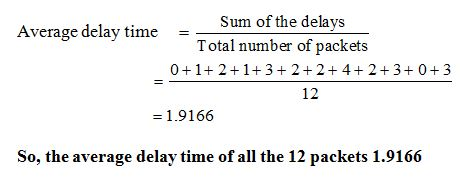C)

Consider the Weighted Fair Queuing and that WFQ service is used, with packets 1, 4, 5, 6, and 11 belonging to one class of traffic, and the remaining packets belonging to the second class of traffic. Class 1 has a WFQ weight of 1, while class 2 has a WFQ weight of 2 .

The following table indicating the arrival time and the time at which the each packet leaves the queue: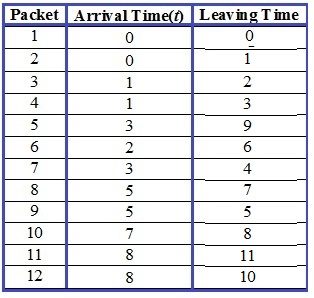The formula for the Delay time  = leaving time – arrival time.

The following table calculate the delay time of the packets by using above formula: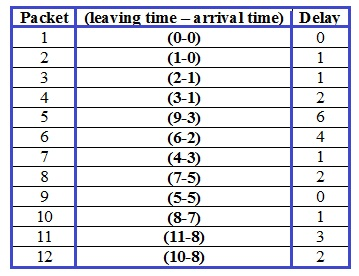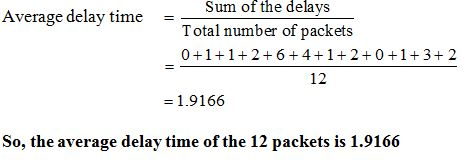### Discussions

Post the discussion to improve the above solution.